Students can Download Maths Chapter 5 Information Processing Intext Questions and Answers, Notes Pdf, Samacheer Kalvi 7th Maths Book Solutions Guide Pdf helps you to revise the complete Tamilnadu State Board New Syllabus and score more marks in your examinations.

## Tamilnadu Samacheer Kalvi 7th Maths Solutions Term 2 Chapter 5 Information Processing Intext Questions

Activity (Text book Page No. 91)

Question 1.
Observe the pattern given below. Continue the pattern for three more steps.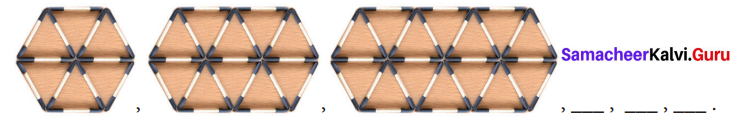Let, ‘x’ be the number of steps and ‘y’ be the number of match sticks. Tabulate the values of ‘x’ and ‘y’ and verify the relationship y = 7x + 5.
Solution:
Three more patterns are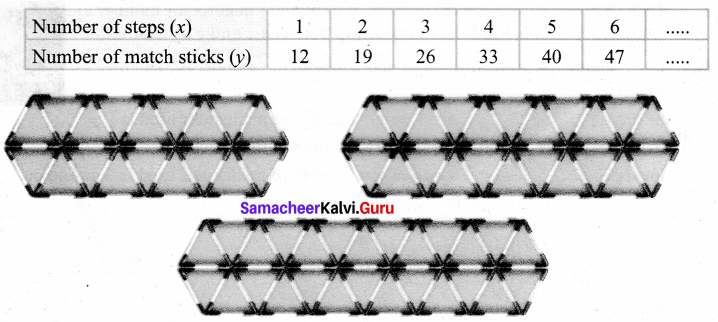From the table y = 7x + 5 is verified.Try These (Text book Page No. 92)

Question 1.
In the given figure, let Y denote the number of steps and y denote its area. Find the relationship between x and y by tabulation.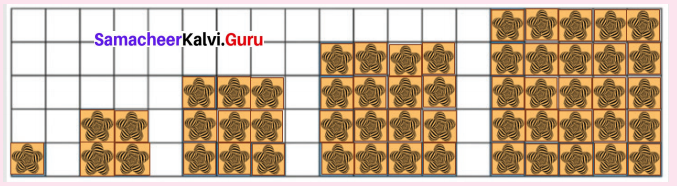Solution:
Let x denote the number of steps and y denote the area.
In the first shape let x = 1 and the area be 1 cm2
when x = 2 : Area = 22 = 4 cm2
whenx = 3 : Area = 32 = 9 cm2 and so on.
Tabulating the values of x and y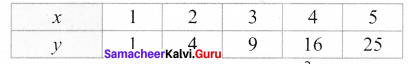From the table:
x = 1 ⇒ y = 12
x = 2 ⇒ y = 22
x = 4 ⇒ y = 42
x = 5 ⇒ y = 52
Hence the relationship between x and y is y = x2Question 2.
In the figure, let x denotes the number of steps and y denotes the number of matchsticks used. Find the relationship between Y and y by tabulation.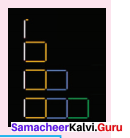Solution:
Let x denote the number of steps and y denote the number of matchsticks used.
In step 1, x = 1 ⇒ y = number of mathsticks used is 1
In step 2, x = 2 ⇒ y = number of mathsticks used is 4
In step 3, x = 3 ⇒ y = number of mathsticks used is 7 and so on.
The values of v andy are tabulated as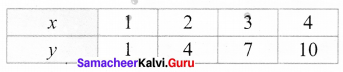x = 1 ⇒ y = 1 = 3(1) – 2
x = 2 ⇒ y = 4 = 3(2) – 2
x = 3 ⇒ y = 7 = 3(3) – 2
x = 4 ⇒ y = 10 = 3(4) – 2
From the table y = 3x – 2Question 3.
Observe the table given below.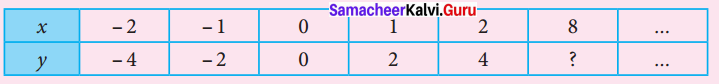Find the relationship between x and y. What will be the value of y, when x = 8.
Solution:
Soil When x = – 2 y = 2 (-2) = -4
When x = -1 y = 2 (-1) = -2
When x = 0 y = 2 (0) = 0
When x =1 y = 2(1) = 2
When x = 2 y = 2 (2) = 4
When x = 8 y = 2 (8) = 16.
Also y = 2x is the relation between x and y.Activity (Text book Page No. 93 & 94)

Question 1.
Complete the following Pascal’s Triangle by observing the number pattern.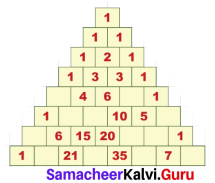Solution:
Pascal’s triangle is given by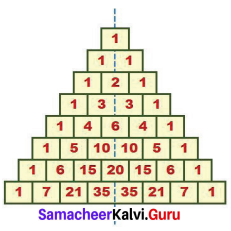Question 2.
Observe the above completed and r find the sequence that you see in it and complete them.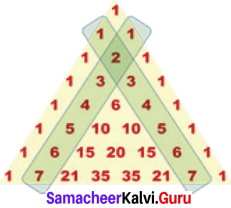(i) 1,2, 3, 4, 5, 6, 7.
(ii) 1, 3, _____, _____, _____, _____.
(iii) 1, _____, _____, _____, _____,
(iv) _____, _____, _____, _____.
Solution:
(i) 1,2, 3, 4, 5, 6, 7.
(ii) 1,3,6,10,15,21.
(iii) 1,4,10,20,35.
(iv) 1,5,15,35.Question 3.
Observe the sequence of numbers obtained in the 3rd and 4th slanting rows of Pascal’s Triangle and find the difference between the consecutive numbers and complete the table given below.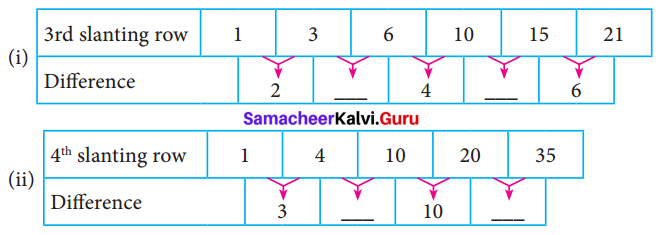Solution: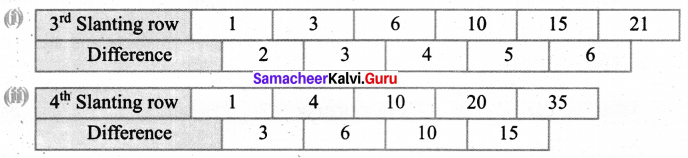Try These (Text book Page No. 96)

Question 1.
Observe the pattern of numbers given in the slanting rows earlier and complete the Pascal’s Triangle.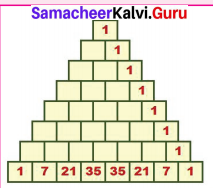Solution: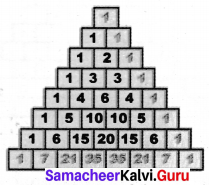Question 2.
Complete the given Pascal’s Triangle. Find the common property of the numbers filled by you. Can you relate this pattern with the pattern discussed in situation 2. Discuss.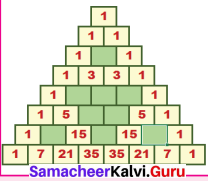Solution: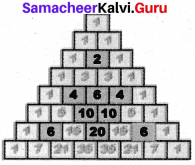Common Properties:
The numbers filled by me are even numbers. Also they make triangular shape.
Yes, this pattern and the pattern given in situation 2 have the same properties.